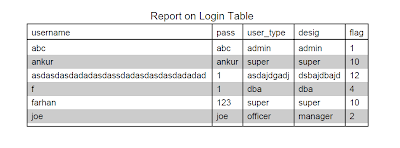## Generating tabulated PDF file from database using CodeIgniter

After my previous post on Generating PDF Files from Database using CodeIgniter, this is post which will teach you how you can generated pdf files with data displayed in a tabular format. Like below :

﻿PDF File with Tabulated Data

The data is taken from MySQL database and then that data is displayed as it appears in the table. This is quiet a difficult task to do, but don't worry i have made this easy enough to understand.

We have already seen that CodeIgniter follows the MC pattern and hence we will not discuss more about CodeIgniter but will directly move on to the point.

# Lets Start

When you are dealing with business reports the odds are good that you will need to generate reports with tables to display tabular data. From my experience with other PDF libraries, this is not an easy task. But with the R&OS library it’s about as hard as creating an array.

```<?php
function create_table(){

\$data = array();
\$query = \$this->db->query('SELECT * FROM <tablename>');
\$num = \$query->num_fields();
\$farr=array();
\$i=1;
\$file_name = 'Products_'.date('dMy').'.pdf';
\$fname="";
while(\$i <= \$num){
\$test = \$i;
\$value = \$this->input->post(\$test);

if(\$value != ''){
\$fname= \$fname." ".\$value;
array_push(\$farr, \$value);

}
\$i++;
}

\$fname = trim(\$fname);

\$fname=str_replace(' ', ',', \$fname);

\$query = 'select '.\$fname.' from <tablename>';
\$result = mysql_query(\$query);

// step through the result set, populating the array,```
``` note that this could also have been written:
// while(\$data[] = mysql_fetch_assoc(\$result)) {}
while(\$data[] = mysql_fetch_array(\$result, MYSQL_ASSOC)) {}
// make the table

// do the output, this is my standard testing output code,```
``` adding ?d=1
// to the url puts the pdf code to the screen in raw form,```
``` good for checking
// for parse errors before you actually try to generate the```
``` pdf file.

if (isset(\$d) && \$d){
\$pdfcode = \$pdf->output(1);
\$pdfcode = str_replace("\n","\n<br>",htmlspecialchars(\$pdfcode));
echo '<html><body>';
echo trim(\$pdfcode);
echo '</body></html>';
} else {
\$pdfcode = \$this->cezpdf->ezOutput();
\$fp = fopen(\$file_name,'wb');
fwrite(\$fp,\$pdfcode);
fclose(\$fp);
}
}
?>
```

The above code produces a pdf file which is same as the above figure.

In the above code i have used mysql_fetch_array() which Returns an array of strings that corresponds to the fetched row, or FALSE if there are no more rows. The type of returned array depends on how result_type is defined. By using MYSQL_BOTH (default), you'll get an array with both associative and number indices. Using MYSQL_ASSOC, you only get associative indices (as mysql_fetch_assoc() works), using MYSQL_NUM, you only get number indices (as mysql_fetch_row() works).

The values which is returned gets stored in the data[] array which is then provided to the R&OS function called ezTable()  which generated the PDF with tabular data in it.

You can read more about ezTable and other R&OS methods by clicking here.Article by Farhan Khwaja Farhan has written 93 articles . If you like This post, you can follow Code 2 Learn on Twitter. Subscribe to Code 2 Learn feed via RSS or EMAIL to receive instant updates. Want to write for Code 2 Learn. Email us :  codeme@code2learn.com

1.2.